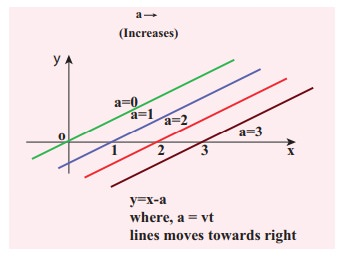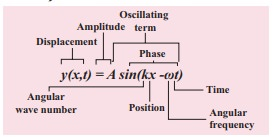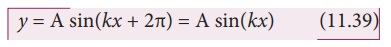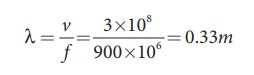Home | | Physics 11th std | Progressive Waves (or) Travelling Waves

# Progressive Waves (or) Travelling Waves

If a wave that propagates in a medium is continuous then it is known as progressive wave or travelling wave.

PROGRESSIVE WAVES (OR) TRAVELLING WAVES

If a wave that propagates in a medium is continuous then it is known as progressive wave or travelling wave.

## Characteristics of progressive waves

1. Particles in the medium vibrate about their mean positions with the same amplitude.

2. The phase of every particle ranges from 0 to 2π.

3. No particle remains at rest permanently. During wave propagation, particles come to the rest position only twice at the extreme points.

4. Transverse progressive waves are characterized by crests and troughs whereas longitudinal progressive waves are characterized by compressions and rarefactions.

5. When the particles pass through the mean position they always move with the same maximum velocity.

6. The displacement, velocity and acceleration of particles separated from each other by are the same, where n is an integer, and λ is the wavelength.

## Equation of a plane progressive waveSuppose we give a jerk on a stretched string at time t = 0s. Let us assume that the wave pulse created during this disturbance moves along positive x direction with constant speed v as shown in Figure 11.23 (a). We can represent the shape of the wave pulse, mathematically as y = y(x, 0) = f(x) at time t = 0s. Assume that the shape of the wave pulse remains the same during the propagation. After some time t, the pulse moving towards the right and any point on it can be represented by x' (read it as x prime) as shown in Figure 11.23 (b). Then,Similarly, if the wave pulse moves towards left with constant speed v, then y = f(x + vt). Both waves y = f(x + vt) and y = f(xvt) will satisfy the following one dimensional differential equation known as the wave equationwhere the symbol ∂ represent partial (read ∂y/∂x as partial y by partial x). Not all the solutions satisfying this differential equation can represent waves, because any physical acceptable wave must take finite values for all values of x and t. But if the function represents a wave then it must satisfy the differential equation. Since, in one dimension (one independent variable), the partial derivative with respect to x is the same as total derivative in coordinate x, we writeThis can be extended to more than one dimension (two, three, etc.). Here, for simplicity, we focus only on the one dimensional wave equation.

### EXAMPLE 11.11

Sketch y = xa for different values of a.

### SolutionThis implies, when increasing the value of a, the line shifts towards right side. For a = vt, y = x vt satisfies the differential equation. Though this function satisfies the differential equation, it is not finite for all values of x and t. Hence, it does not represent a wave.

### EXAMPLE 11.12

How does the wave y = sin(xa) for a = 0, a = π/4, a = π/2, a = 3π/2, a = π look like?. Sketch this wave.

### SolutionFrom the above picture we observe that y = sin (x−a) for a = 0, a = π/4, a = π/2, a = 3π/2 and a = π, the function y = sin (x−a) shifts towards right. Further, we can take a = vt and v = π/4 ,  and sketching for different times t = 0s, t = 1 s, t = 2s etc., we once again observe that y = sin(xvt) moves towards the right. Hence, y = sin(xvt) is a travelling (or progressive) wave moving towards the right. If y = sin(x+vt) then the travelling (or progressive) wave moves towards the left. Thus, any arbitrary function of type y = f(xvt) characterising the wave must move towards right and similarly, any arbitrary function of type y = f(x+vt) characterizing the wave must move towards left.

### EXAMPLE 11.13

Check the dimensional of the wave y = sin(xvt). If it is dimensionally wrong, write the above equation in the correct form.

### Solution

Dimensionally it is not correct. we know that y = sin(xvt) must be a dimensionless quantity but xvt has dimension. The correct equation is y = sin (k xωt), where k and ω have the dimensions of inverse of length and inverse of time respectively. The sine functions and cosine functions are periodic functions with period 2π. Therefore, the correct expression is y = sin ([2π/λ]x, [2π/T]t) where λ and T are wavelength and time period, respectively.

In general, y(x,t)=A sin(k xωt).## Graphical representation of the wave

Let us graphically represent the two forms of the wave variation

(a)  Space (or Spatial) variation graph

(b)  Time (or Temporal) variation graph

### (a)  Space variation graphBy keeping the time fixed, the change in displacement with respect to x is plotted. Let us consider a sinusoidal graph, y = A sin(kx) as shown in the Figure 11.24, where k is a constant. Since the wavelength λ denotes the distance between any two points in the same state of motion, the displacement y is the same at both the ends y = x and y = x + λ, i.e.,The sine function is a periodic function with period 2π. Hence,Comparing equation (11.38) and equation (11.39), we get

kx + k λ = kx + 2π

This implieswhere k is called wave number. This measures how many wavelengths are present in 2π radians.

The spatial periodicity of the wave is

Then,

At t = 0 s     y(x, 0) = y(x + λ, 0)

and

At any time t, y(x, t) = y(x + λ, t)

### EXAMPLE 11.14

The wavelength of two sine waves are λ1 = 1m and λ2 = 6m. Calculate the corresponding wave numbers.

### Solution### (b) Time variation graphBy keeping the position fixed, the change in displacement with respect to time is plotted. Let us consider a sinusoidal graph, y =A sin(ωt) as shown in the Figure 11.25, where ω is angular frequency of the wave which measures how quickly wave oscillates in time or number of cycles per second.

The temporal periodicity or time period isThe angular frequency is related to frequency f by the expression ω = 2 πf, where the frequency f is defined as the number of oscillations made by the medium particle per second. Since inverse of frequency is time period, we have,This is the time taken by a medium particle to complete one oscillation. Hence, we can define the speed of a wave (wave speed, v) as the distance traversed by the wave per secondwhich is the same relation as we obtained in equation (11.4).

## Particle velocity and wave velocity

In a plane progressive harmonic wave, the constituent particles in the medium oscillate simple harmonically about their equilibrium positions. When a particle is in motion, the rate of change of displacement at any instant of time is defined as velocity of the particle at that instant of time. This is known as particle velocity.Similarly, we can define velocity (here speed) for the travelling wave (or progressive wave). In order to determine the velocity of a progressive wave, let us consider a progressive wave (shown in Figure 11.23) moving towards right. This can be mathematically represented as a sinusoidal wave. Let P be any point on the phase of the wave and yP be its displacement with respect to the mean position. The displacement of the wave at an instant t is

y = y(x,t) = A sin(k x ω t)

At the next instant of time = t + ∆t the position of the point P is = x + ∆x. Hence, the displacement of the wave at this instant isSince the shape of the wave remains the same, this means that the phase of the wave remains constant (i.e., the y- displacement of the point is a constant). Therefore, equating equation (11.42) and equation (11.44), we get

y(x',t') = y(x,t), which implies

A sin[k (x + ∆x)− ω (t + ∆t)]= A sin(k xω t)

OrOn simplification of equation (11.45), we getwhere vp is called wave velocity or phase velocity.

By expressing the angular frequency and wave number in terms of frequency and wave length, we obtain### EXAMPLE 11.15

A mobile phone tower transmits a wave signal of frequency 900MHz. Calculate the length of the waves transmitted from the mobile phone tower.

### Solution

Frequency, f = 900 MHz = 900 ×106 Hz

The speed of wave is c = 3 × 108m s−1Study Material, Lecturing Notes, Assignment, Reference, Wiki description explanation, brief detail
11th Physics : UNIT 11 : Waves : Progressive Waves (or) Travelling Waves |